Algebra 1 2-6 Complete Lesson: Ratios, Rates, and Conversions
starstarstarstarstarstarstarstarstarstar
5 (1 rating)
by Matthew Richardson
| 32 Questions
Note from the author:
A complete formative lesson with embedded slideshow, mini lecture screencasts, checks for understanding, practice items, mixed review, and reflection. I create these assignments to supplement each lesson of Pearson's Common Core Edition Algebra 1, Algebra 2, and Geometry courses. See also mathquest.net and twitter.com/mathquestEDU.The outlined content above was added from outside of Formative.1
2
1
10 pts
Solve It! Two olympic athletes can run the races in the times shown. Who is the faster runner?
Athlete A
Athlete B
2
10 pts
Solve It! How do you know? Explain your reasoning.3
3
10 pts
Vocabulary: What is the ratio of blue to red flowers?
Select all that apply.
4/3
3 : 4
3/4
3 to 4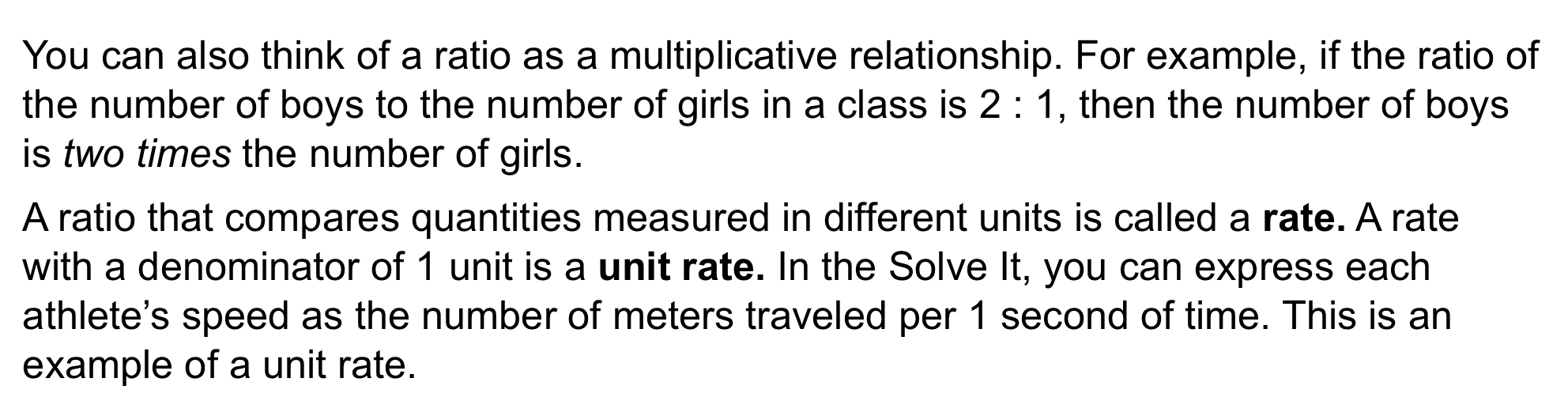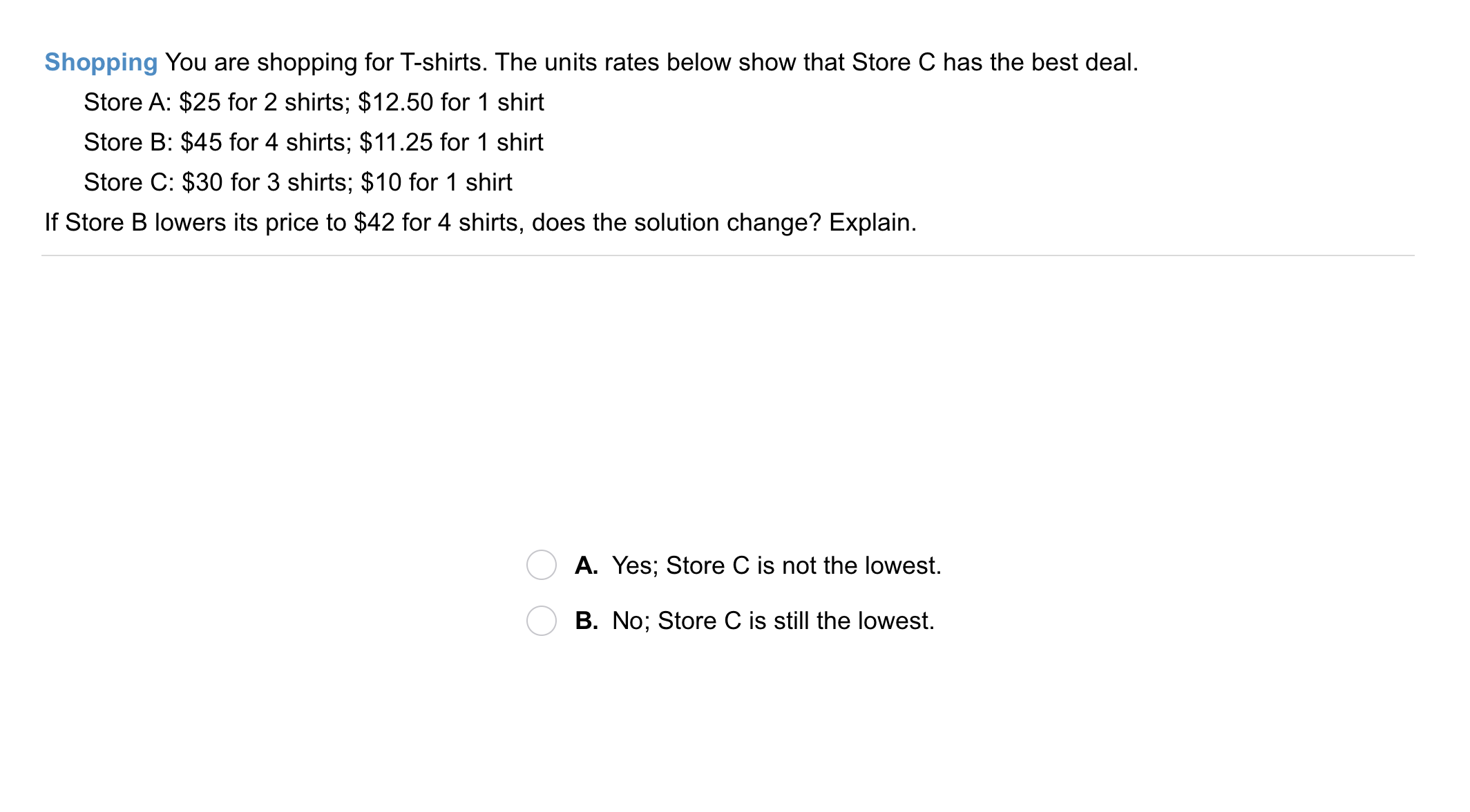5
5
10 pts
Problem 1 Got It?
A
B7
7
10 pts
Problem 2 Got It?
A
B
C
D8
10 pts
Vocabulary: Define unit analysis in your own words. You may include examples, but remember that examples are NOT definitions.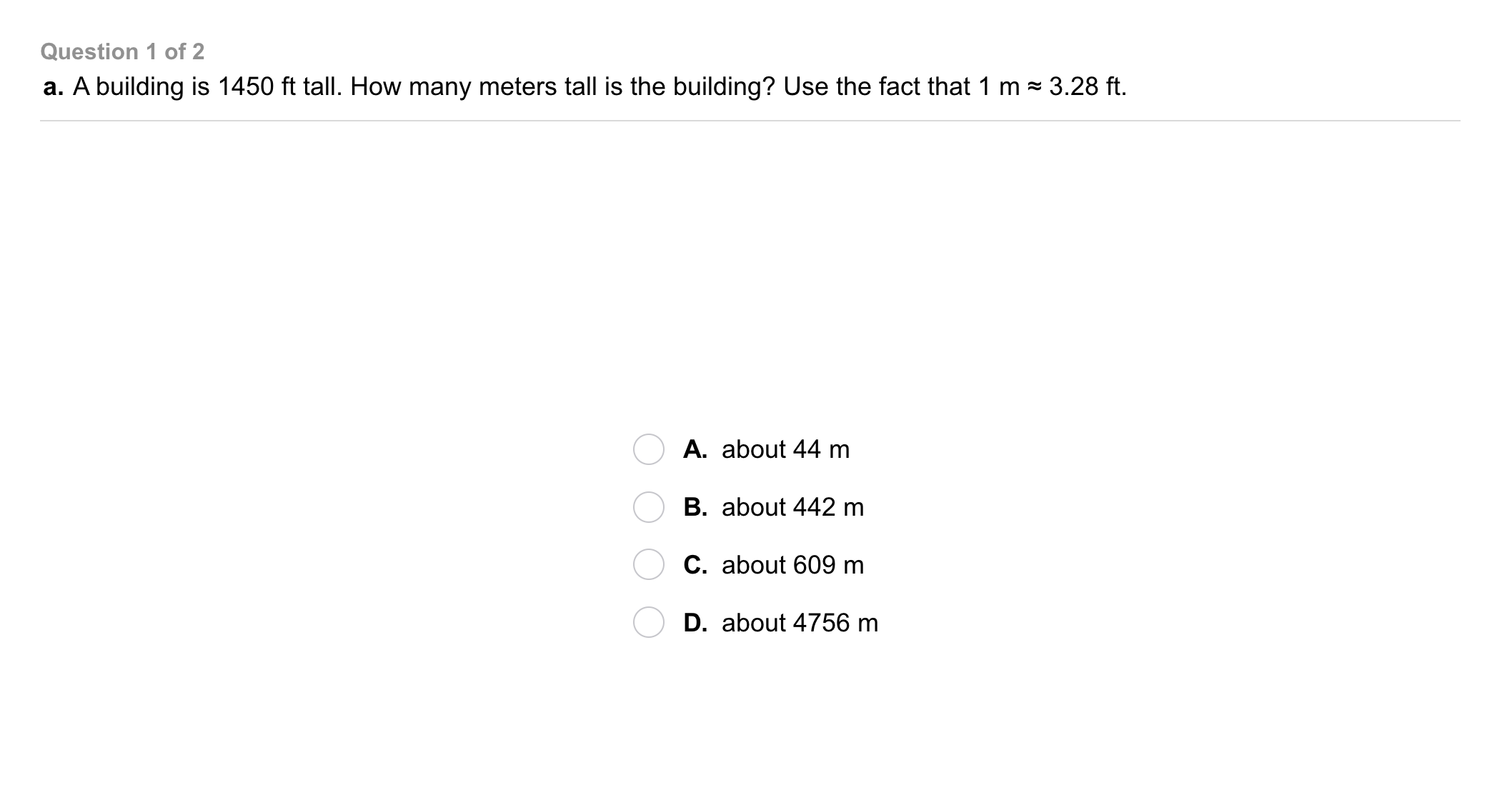9
9
10 pts
Problem 3 Got It?
A
B
C
D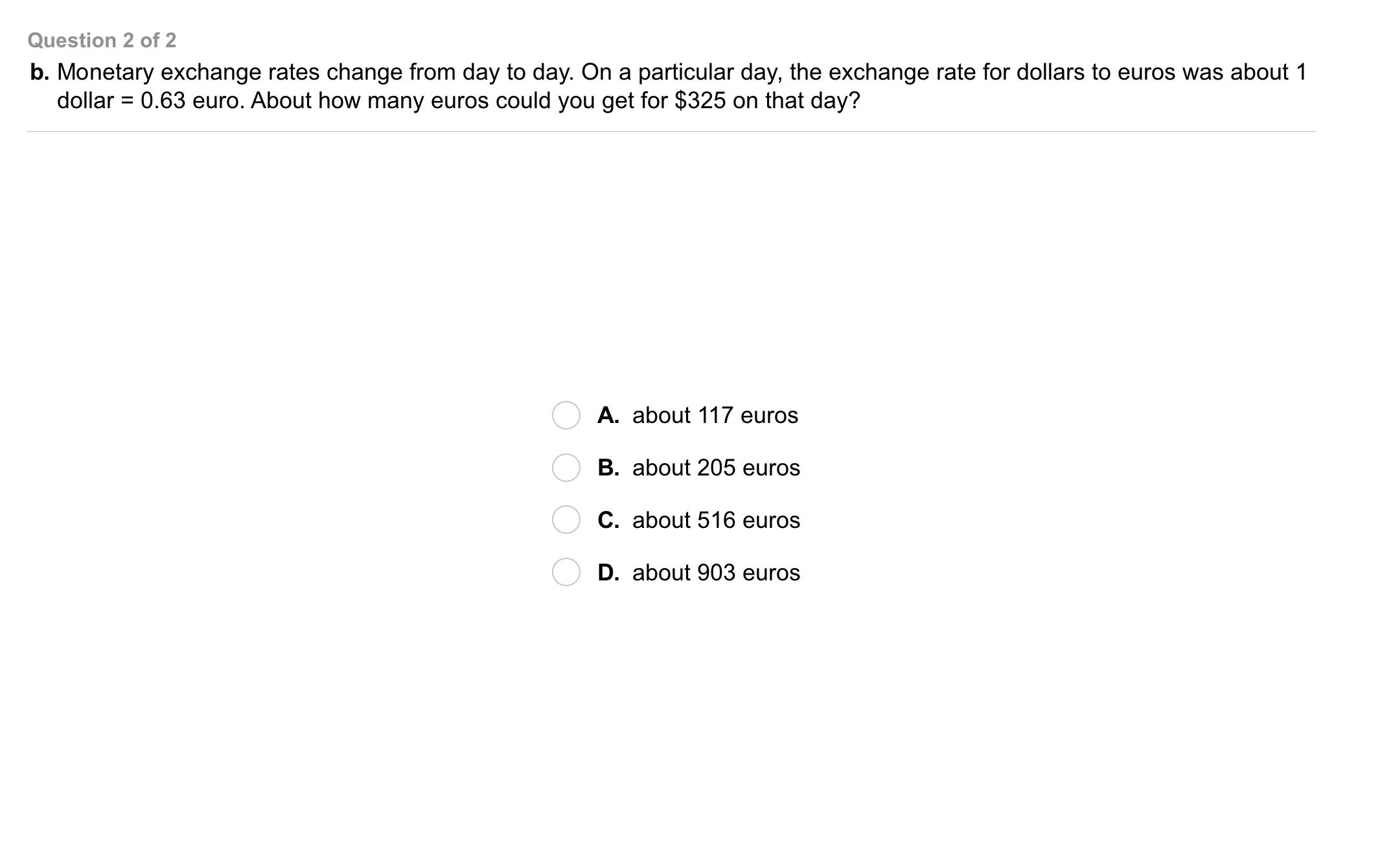10
10
10 pts
Problem 3 Got It?
A
B
C
D
The outlined content above was added from outside of Formative.11
10 pts
Problem 4 Got It? An athlete ran a sprint of 100 ft in 3.1 s. At what speed was the athlete running in miles per hour? Round to the nearest mile per hour. Enter only a number.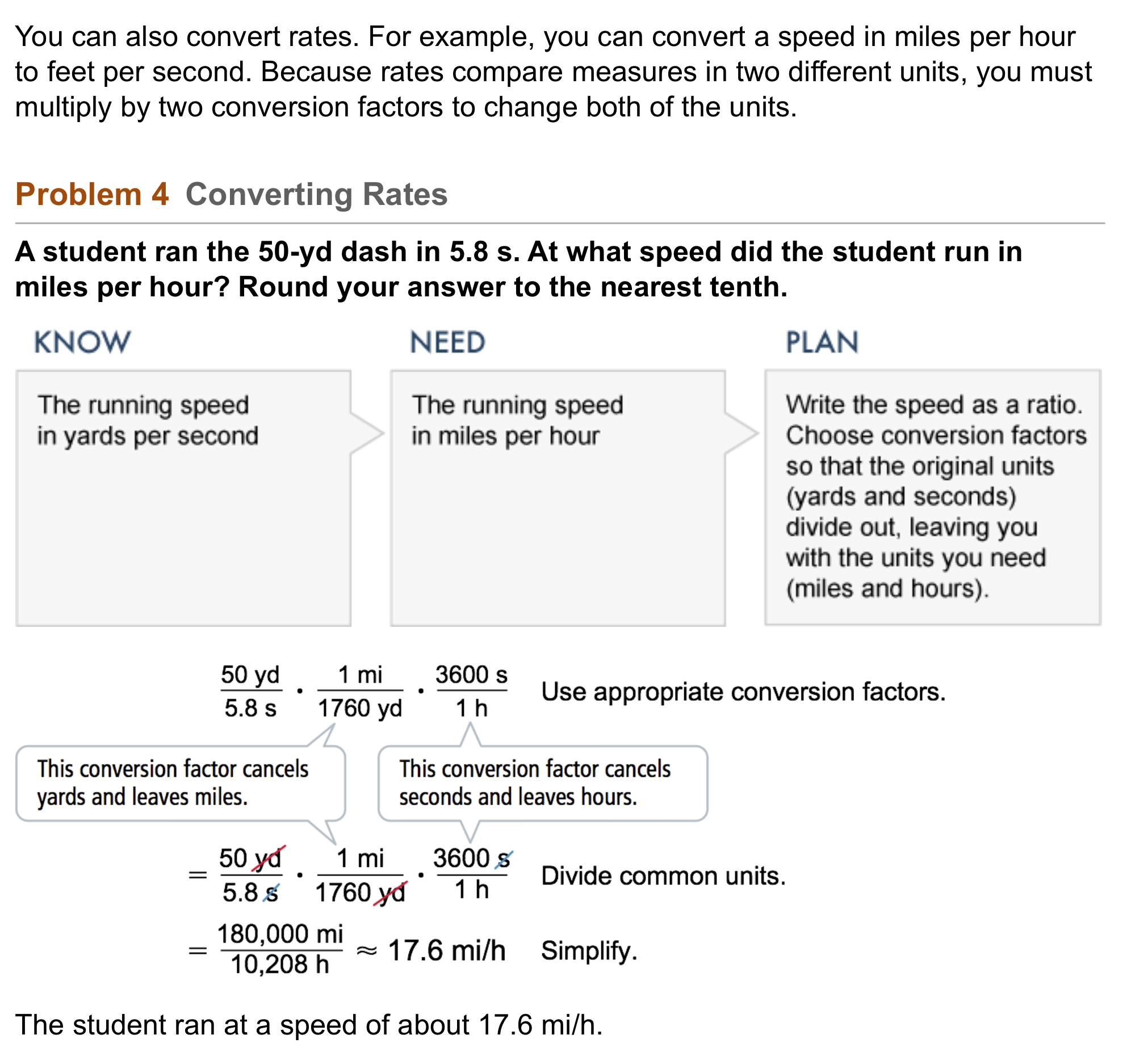1213
13
10 pts
A
B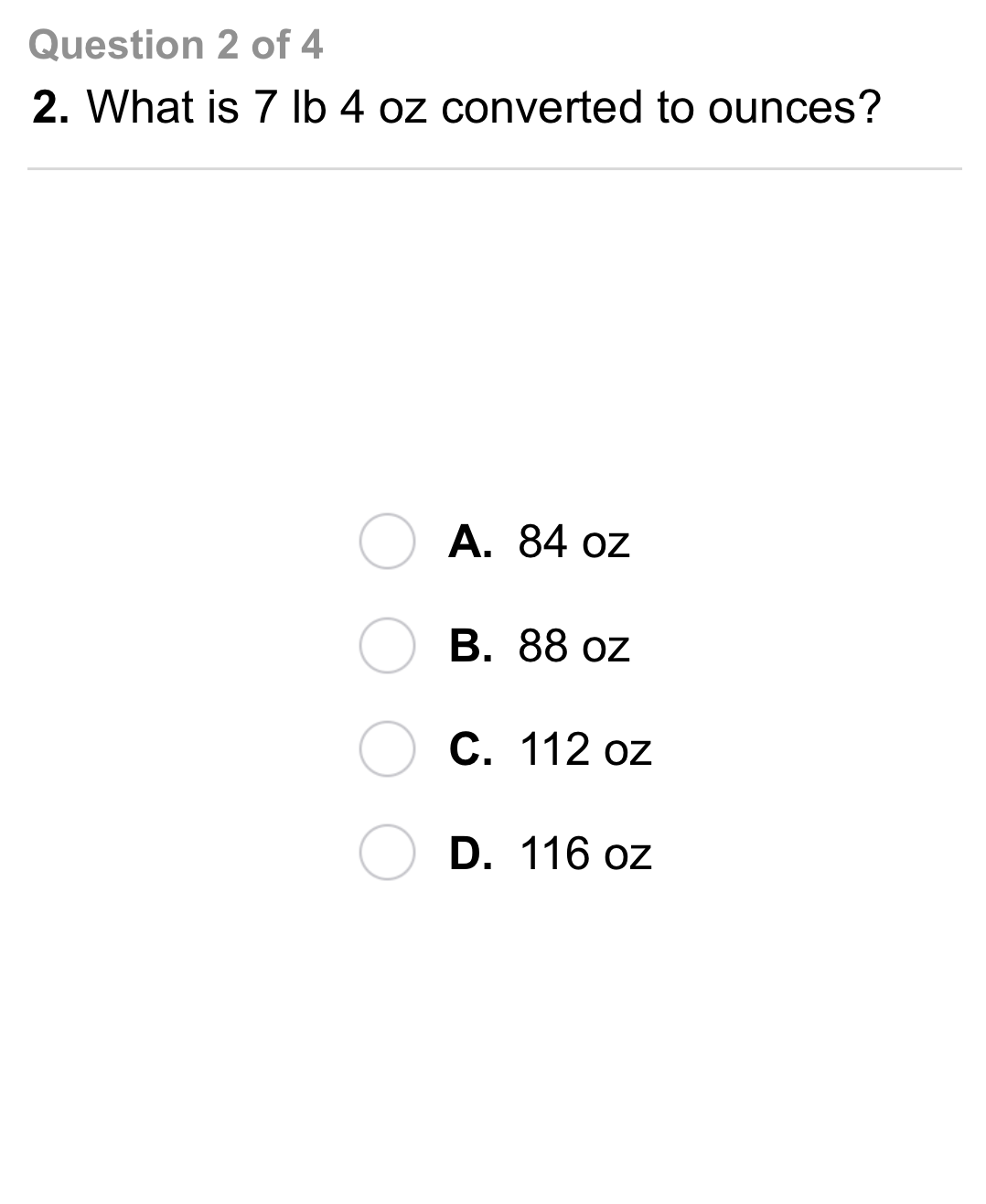14
14
10 pts
A
B
C
D15
15
10 pts
A
B16
16
10 pts
A
B
C
D17
10 pts
Vocabulary: Is this a unit rate?

20 mi every 3 h
Yes
No
18
10 pts
Vocabulary: Is this a unit rate?

2 dollars per day
Yes
No
19
10 pts
Reasoning: Does multiplying by a conversion factor change the amount of what is being measured? How do you know?
20
10 pts
Reasoning: If you convert pounds to ounces, will the number of ounces be greater or less than the number of pounds? Explain.21
10 pts
Review Lesson 2-5: What is the height of a triangle with an area of 30 cm² and a base length of 12 cm?
3 cm
5 cm
10 cm
15 cm
22
10 pts
Review Lesson 2-3: Solve the equation. Check your answer.
Enter only a number.
23
10 pts
Review Lesson 2-3: Solve the equation. Check your answer.
Enter only a number.
24
10 pts
Review Lesson 1-4: Consider the expression.
Identify the following:
1. The step(s) necessary to simplify the expression.
2. The property or properties that justify the simplification.
3. The simplest form of the expression.
• Division property of equality
• 20x
• Divide out x from the numerator and denominator
• 27
• Divide out y from the numerator and denominator

• Step(s) necessary to simplify the expression
• Property or properties that justify the simplification
• Simplest form of the expression
25
10 pts
Review Lesson 1-4: Consider the expression.
Identify the following:
1. The step(s) necessary to simplify the expression.
2. The property or properties that justify the simplification.
3. The simplest form of the expression.
• Division property of equality
• 20x
• Divide out x from the numerator and denominator
• 27
• Divide out y from the numerator and denominator

• Step(s) necessary to simplify the expression
• Property or properties that justify the simplification
• Simplest form of the expression
26
10 pts
Review Lesson 1-4: Consider the expression.
Identify the following:
1. The step(s) necessary to simplify the expression.
2. The property or properties that justify the simplification.
3. The simplest form of the expression.
• Division property of equality
• 20x
• Divide out x from the numerator and denominator
• 27
• Divide out y from the numerator and denominator

• Step(s) necessary to simplify the expression
• Property or properties that justify the simplification
• Simplest form of the expression27
10 pts
Vocabulary Review: Write a fraction with a numerator of 12 and a denominator of 13.
28
10 pts
Vocabulary Review: Classify the fractions on the left based on whether or not they are in simplest form.

• In simplest form
• NOT in simplest form
29
10 pts
Vocabulary Review: What is the greatest common divisor of the numerator and denominator of a fraction that is in simplest form.
0
1
2
3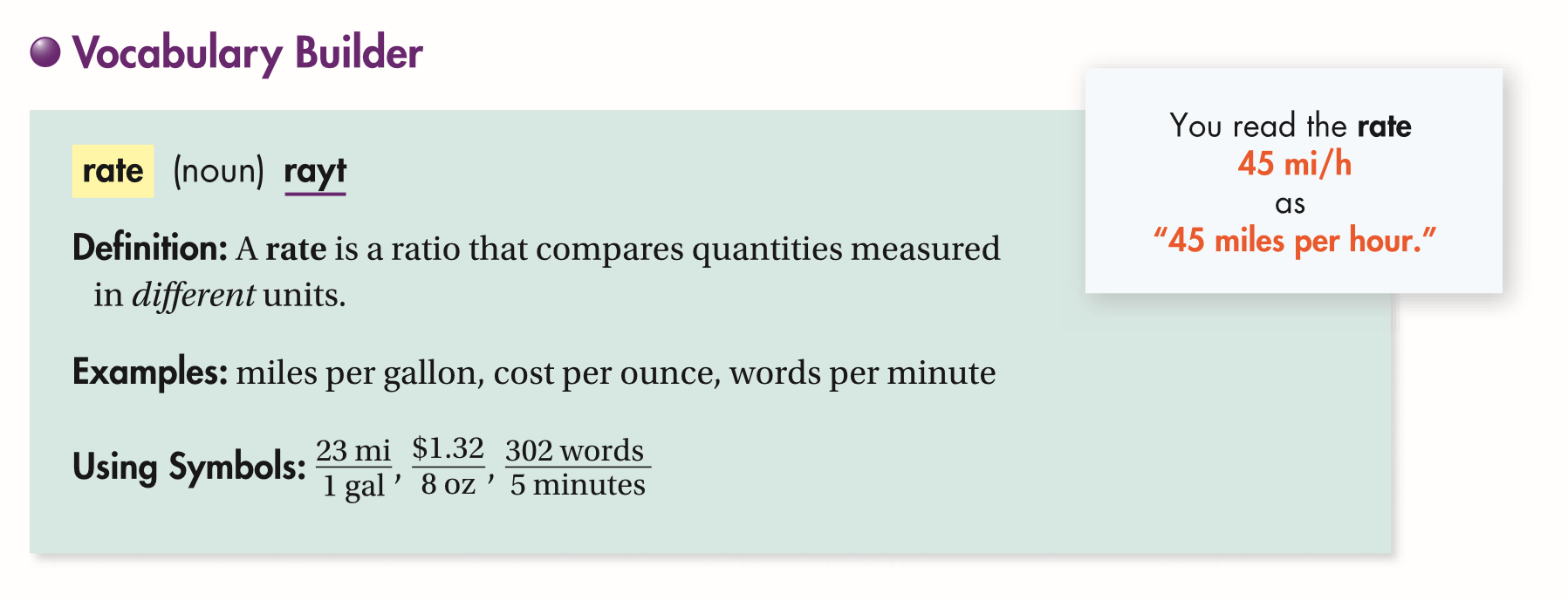30
10 pts
Use Your Vocaulary: Match each rate on the left with the situation it describes on the right.
• 20 mph
• 20 bagels / 3 hours
• A bakery makes 20 bagels every 3 hours.
• Chandler bicycles 20 miles each hour.
31
100 pts
Notes: Take a clear picture or screenshot of your Cornell notes for this lesson. Upload it to the canvas. Zoom and pan as needed.

For a refresher on the Cornell note-taking system, click here.
32
10 pts
Reflect: Math Success
Add to my formatives list

Formative uses cookies to allow us to better understand how the site is used. By continuing to use this site, you consent to the Terms of Service and Privacy Policy.